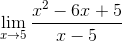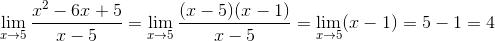# Calculus 2 : Finding Limits and One-Sided Limits

## Example Questions

← Previous 1 3 4 5 6 7 8 9 41 42

### Example Question #1 : Finding Limits And One Sided Limits

Which graph is a possible sketch of the functionthat possesses the following characteristics: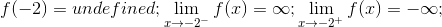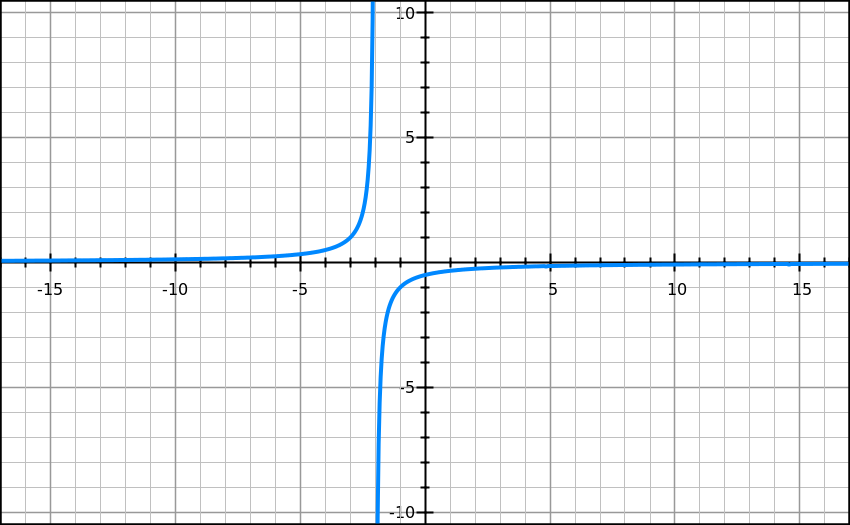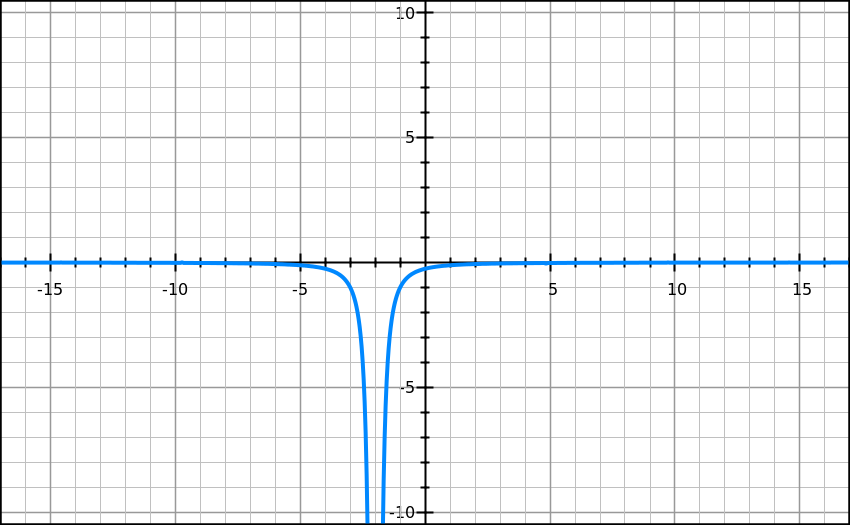There does not exist such a graph.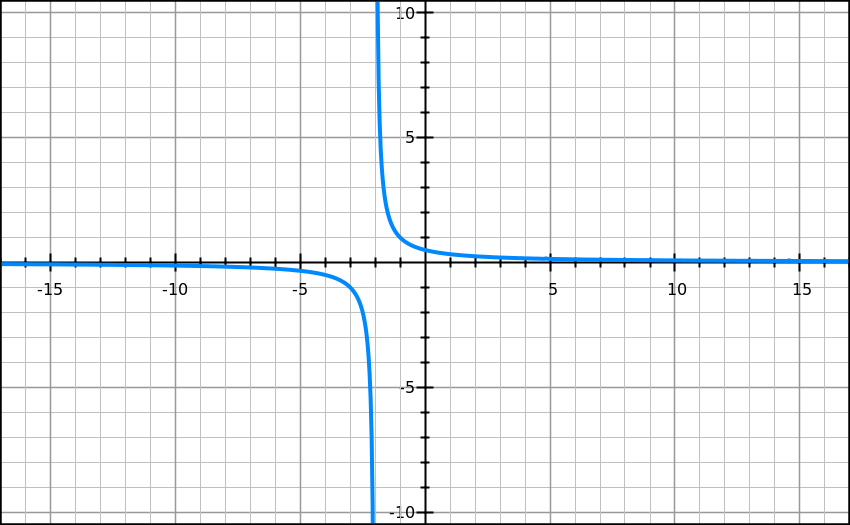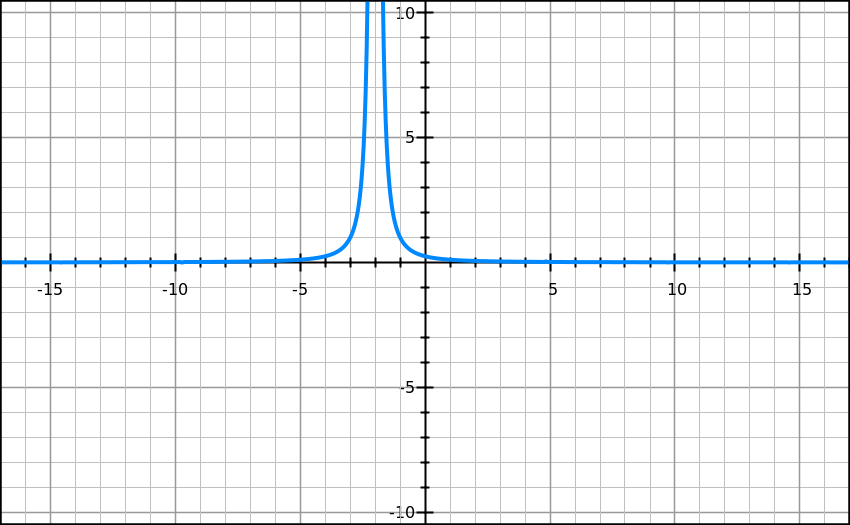Explanation:

Since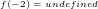there is a possible vertical asymptote at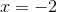.

As we approachfrom the left, the graph should tend to. Approachingfrom the right, the graph tends to.

The only graph that does so is.

### Example Question #2 : Finding Limits And One Sided Limits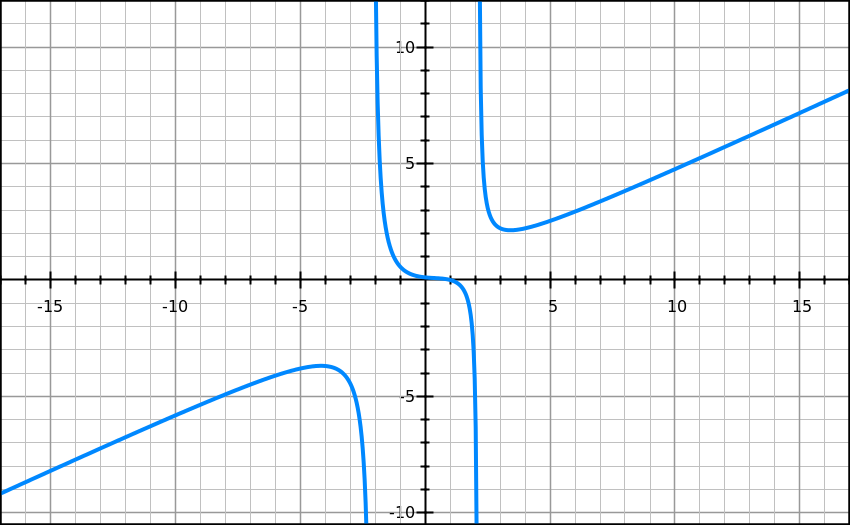The graph above is a sketch of the function. Find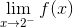.Does not exist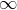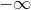Explanation:

We need to look at the behavior of the function as it tends tofrom the left.  Therefore the answer is### Example Question #3 : Finding Limits And One Sided LimitsThe graph above is a sketch of. Find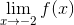.Does not existDoes not exist

Explanation:

The limit does not exist because the one-sided limits are not equal;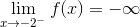, whereas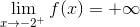### Example Question #4 : Finding Limits And One Sided Limits

Determine the value of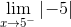.Explanation:

The expressionindicates that all points on the domain are equal to 5 since the absolute value negates negative values.

The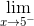is to search for the limit as the graph approachesfrom the left side of the graph.  Since the absolute value of negative five is five, the graph approaches five from the left.

The correct answer is.

### Example Question #5 : Finding Limits And One Sided Limits

Evaluate the following limit.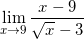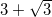Limit does not existExplanation:

This limit can be solved using simple manipulation of the expression inside the limit: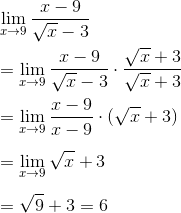### Example Question #6 : Finding Limits And One Sided Limits

Evaluatle the limit: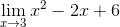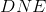Explanation:

Consider the domain of the function. Because this equation is a polynomial, x is not restricted by any value. Thus the way to evaluate this limit would simply be to plug the value that x is approaching into the limit equation.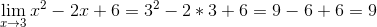### Example Question #7 : Finding Limits And One Sided Limits

Evaluate the limit: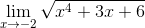Explanation:

Consider the domain of the function. Because this equation is a polynomial, x is not restricted by any value. Thus the way to evaluate this limit would simply be to plug the value that x is approaching into the limit equation.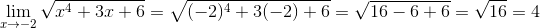### Example Question #1 : Finding Limits And One Sided Limits

Evaluate the limit: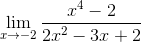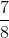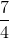Explanation:

The limiting situation in this equation would be the denominator. Plug the value that x is approaching into the denominator to see if the denominator will equal 0. In this question, the denominator will not equal zero when x=-2; so we proceed to insert the value of x into the entire equation.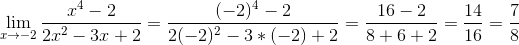### Example Question #9 : Finding Limits And One Sided Limits

Evaluate the limit: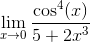Explanation:

The limiting situation in this equation would be the denominator. Plug the value that x is approaching into the denominator to see if the denominator will equal 0. In this question, the denominator will not equal zero when x=0; so we proceed to insert the value of x into the entire equation.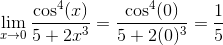### Example Question #10 : Finding Limits And One Sided Limits

Evaluate the limit: